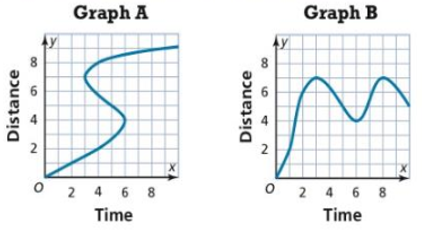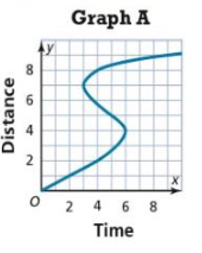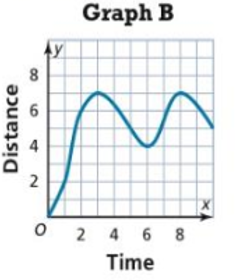Maths-
General
Easy

Question

#Tell whether each graph is a function and justify your answer. Which graph is not a good representation of a real world situation? Explain.

## The correct answer is: 2, 6 and 8

### Hint:An equation of the form y = f(x) is called a function if there is a unique value of y for every value of x. In other words, every value of x must have one value of y. We can check if the graph is a function by the vertical line test. A graph represents a function if any vertical line in the xy plane cuts the graph at maximum one point.Step by step solution:The first graph isWe check if the graph is a function by vertical line test.When we draw a vertical line at x = 4, it cut the graph at 3 points.Thus, graph A is not a function.Graph B isWe can observe that any vertical line on the plane cuts the graph at maximum one point.Thus, the above graph is a function.In graph A, at time t = 4, we can see that d has three values, which are 2, 6 and 8.This means that at the same time t=4, the distances travelled are 2, 6 and 8, which is physically impossible. There can be only one value of distance associated with a value of time which is the case in graph B.Thus, graph A is not a good example of a real world situation.Note:In a function, every value of x must have one value of y. When we draw a vertical, it cuts x axis at one point, we take that point to be x. If the vertical line cuts the graph at two points, then it gives two values of y for one value of x. This does not represent a function.#### With Turito Foundation.#### Get an Expert Advice From Turito.